# Difference between revisions of "Curvature is tensorial"

This article gives the statement, and possibly proof, that a map constructed in a certain way is tensorial
View other such statements

## Statement

Let$\nabla$ be a connection on a vector bundle$E$ over a differential manifold$M$. The Riemann curvature tensor of$\nabla$ is given as a map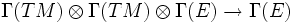$\Gamma(TM) \otimes \Gamma(TM) \otimes \Gamma(E) \to \Gamma(E)$ defined by: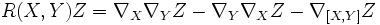$R(X,Y)Z = \nabla_X\nabla_Y Z - \nabla_Y \nabla_X Z - \nabla_{[X,Y]} Z$

We claim that$R$ is a tensorial map in each of the variables$X,Y,Z$.

## Facts used

• Leibniz rule for derivations: This states that for a vector field$X$ and functions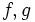$f,g$, we have: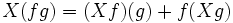$X(fg) = (Xf)(g) + f(Xg)$

• Corollary of Leibniz rule for Lie bracket: This states that for a function$f$ and vector fields$X,Y$: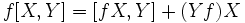$f[X,Y] = [fX,Y] + (Yf)X$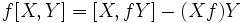$f[X,Y] = [X,fY] - (Xf)Y$

• The Leibniz rule axiom that's part of the definition of a connection, namely: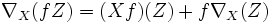$\nabla_X(fZ) = (Xf)(Z) + f\nabla_X(Z)$

## Proof

To prove tensoriality in a variable, it suffices to show$C^\infty$-linearity in that variable. This is because linearity in$C^\infty$-functions guarantees linearity in a function that is 1 at exactly one point, and zero at others.

The proofs for$X$ and$Y$ are analogous, and rely on manipulation of the Lie bracket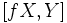$[fX,Y]$ and the property of a connection being$C^\infty$ in the subscript vector. These proofs do not involve any explicit use of$Z$. The proof for$Z$ relies simply on repeated application of the product rule, and the fact that$XY - YX = [X,Y]$.

### Tensoriality in the first variable

Let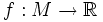$f:M \to \R$ be a scalar function. We will show that: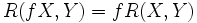$\! R(fX,Y) = f R(X,Y)$

We start out with the left side: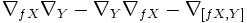$\nabla_{fX}\nabla_Y - \nabla_Y \nabla_{fX} - \nabla_{[fX,Y]}$

Now by the definition of a connection,$\nabla$ is$C^\infty$-linear in its subscript argument. Thus, the above expression can be written as: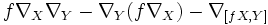$f\nabla_X\nabla_Y - \nabla_Y (f \nabla_X) - \nabla_{[fX,Y]}$

Now applying the Leibniz rule for connections, we get: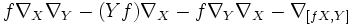$f\nabla_X\nabla_Y - (Yf)\nabla_X - f \nabla_Y\nabla_X - \nabla_{[fX,Y]}$

We can rewrite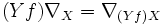$(Yf)\nabla_X = \nabla_{(Yf)X}$ and we then get: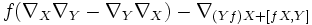$f(\nabla_X\nabla_Y - \nabla_Y\nabla_X) - \nabla_{(Yf)X + [fX,Y]}$

By the corollary stated above, we have: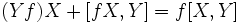$\! (Yf)X + [fX,Y] = f[X,Y]$

which, substituted back, gives: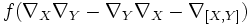$f(\nabla_X\nabla_Y - \nabla_Y\nabla_X - \nabla_{[X,Y]})$

### Tensoriality in the second variable

Let$f:M \to \R$ be a scalar function. We will show that: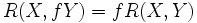$\! R(X,fY) = f R(X,Y)$

We start out with the left side: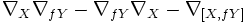$\nabla_X\nabla_{fY} - \nabla_{fY}\nabla_X - \nabla_{[X,fY]}$

Applying the Leibniz rule and the property of a connection being$C^\infty$ in its subscript variable yields:$(Xf)\nabla_Y + f(\nabla_X\nabla_Y - \nabla_Y\nabla_X) - \nabla_{[X,fY]}$

which simplifies to: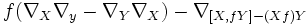$f(\nabla_X\nabla_y - \nabla_Y\nabla_X) - \nabla_{[X,fY] - (Xf)Y}$

We now use the corollary stated above:$\! f[X,Y] = [X,fY] - (Xf)Y$

substituting this gives: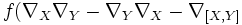$f (\nabla_X\nabla_Y - \nabla_Y\nabla_X - \nabla_{[X,Y]}$

which is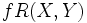$f R(X,Y)$

### Tensoriality in the third variable

Let$f: M \to \R$ be a scalar function. We will show that:$\! R(X,Y) (fZ) = f R(X,Y) Z$

We start out with the left side: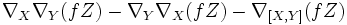$\nabla_X\nabla_Y(fZ) - \nabla_Y\nabla_X(fZ) - \nabla_{[X,Y]}(fZ)$

Now we apply the Leibniz rule for connnections on each term: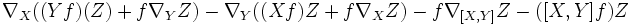$\nabla_X( (Yf)(Z) + f \nabla_YZ) - \nabla_Y ((Xf)Z + f \nabla_XZ) - f \nabla_{[X,Y]}Z - ([X,Y]f) Z$

We again apply the Leibniz rule to the first two term groups: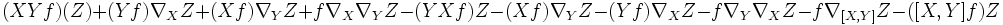$(XYf)(Z) + (Yf) \nabla_XZ + (Xf) \nabla_YZ + f \nabla_X\nabla_YZ - (YXf)Z - (Xf) \nabla_YZ - (Yf) \nabla_XZ -f \nabla_Y\nabla_XZ - f \nabla_{[X,Y]}Z - ([X,Y]f) Z$

After cancellations we are left with the following six terms:$f (\nabla_X\nabla_Y - \nabla_Y\nabla_X - \nabla_{[X,Y]})Z + ((XY - YX - [X,Y])f)Z$

But since$[X,Y] = XY - YX$, the last three terms vanish, and we are left with: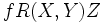$\! f R(X,Y)Z$